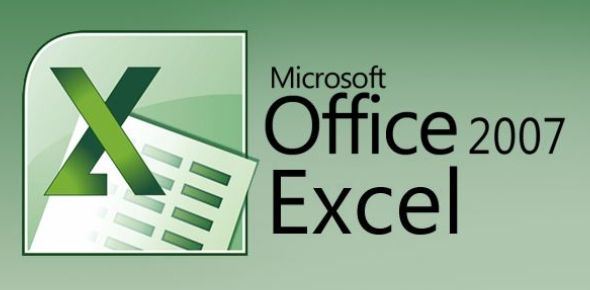# Microsoft Excel 2007 Quiz: Practice Exam!

31 Questions | Total Attempts: 1960SettingsAre you familiar with Microsoft Excel 2007, and would you be interested in answering these questions? In the 2007 version, you can learn how to create a simple budget spreadsheet. You can also format and write formulas. Microsoft Excel includes applications such as Word, Excel, PowerPoint, and Outlook. The application download includes the popular components of each of these programs. If you want to learn more about Microsoft Excel 2007, put your knowledge to the test.

• 1.
Reference to the above image of Command Group "Alignment" which of the following tabs consist it:
• A.

View

• B.

Formatting

• C.

Home

• 2.
You can have results of any database table into Excel Worksheet, and by one click you can refresh the results?
• A.

True

• B.

False

• 3.
What is the maximum number of rows that can be entered on a single Excel 2007 worksheet?
• A.

65536

• B.

Over 1 million

• C.

64000

• D.

256

• 4.
When using a databar, changing the value of one cell in the range will not affect the size of all the other cells.
• A.

True

• B.

False

• 5.
The Insert Function dialog box tells you how to use functions.
• A.

True

• B.

False

• 6.
When a worksheet is printed, the grid lines that surround the cells are printable by default.
• A.

True

• B.

False

• 7.
When you clear a filter, all filters in the worksheet are cleared, if worksheet contains more than one filters
• A.

True

• B.

False

• 8.
The function arguments dialog box tells you how to use functions.
• A.

True

• B.

False

• 9.
The simplest and most convenient way to add a record to a table is to enter the data in the first blank row.
• A.

True

• B.

False

• 10.
If you insert a new row at the top of the table, the new row becomes the header row and is formatted with the table style.
• A.

True

• B.

False

• 11.
In Excel, charts do not remain linked or connected to the data sources if they appear in different worksheets.
• A.

True

• B.

False

• 12.
After you merge a range into a single cell, you can't realign its content.
• A.

True

• B.

False

• 13.
Once you hide a row, it remains hidden as long as the workbook is active.
• A.

True

• B.

False

• 14.
All functions have arguments.
• A.

True

• B.

False

• 15.
Although 3D Charts are visually attractive, they can obscure the relationship between the values in the chart by making it difficult to see when slice is larger.
• A.

True

• B.

False

• 16.
Excel has a builtin format to display 4 digit year values, so you do not need to create on.
• A.

True

• B.

False

• 17.
Formatting data only change the appearance of data, it does not affect the data itself.
• A.

True

• B.

False

• 18.
If a formula contains several functions, Excel starts with the outermost function and then moves inward.
• A.

True

• B.

False

• 19.
An easy way to sort data when there is only one sort field is to use the Sort A to Z or Sort Z to A buttons.
• A.

True

• B.

False

• 20.
You can create maximum one Excel table in a worksheet
• A.

True

• B.

False

• 21.
As you begin to type a function name within a formula into a cell, a list of functions that begin with the letters you typed appears.
• A.

True

• B.

False

• 22.
You can rotate a 3D chart in only two directions: horizontally along the x-axis and vertically along the y-axis.
• A.

True

• B.

False

• 23.
Pressing ENTER moves the selection one cell to the right.
• A.

True

• B.

False

• 24.
Ctrl+B key makes the shortcut for making font in selected cell bold.
• A.

True

• B.

False

• 25.
What key should be used to edit the content of a cell?
• A.

F1

• B.

F2

• C.

F3

Related TopicsBack to top